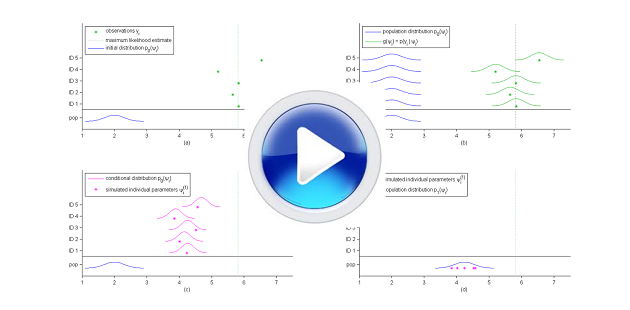# Difference between revisions of "Animations & Videos"

## Introduction to the population approach

The objective of this animation is to explain that such approach is relevant in many applications (biology, agronomy, toxicology, pharmacology,…) and to show a little bit more in detail an application in PK modeling.## Introduction to PK modeling

This animation describes how complex biological phenomena can be approximated by simplified models represented by mathematical equations.Examples on PK modeling using \$\mlxplore\$ can be visualized here.

## The SAEM algorithm in practice

This video shows the behavior of the SAEM algorithm, that is largely decribed in The SAEM algorithm for estimating population parameters section.